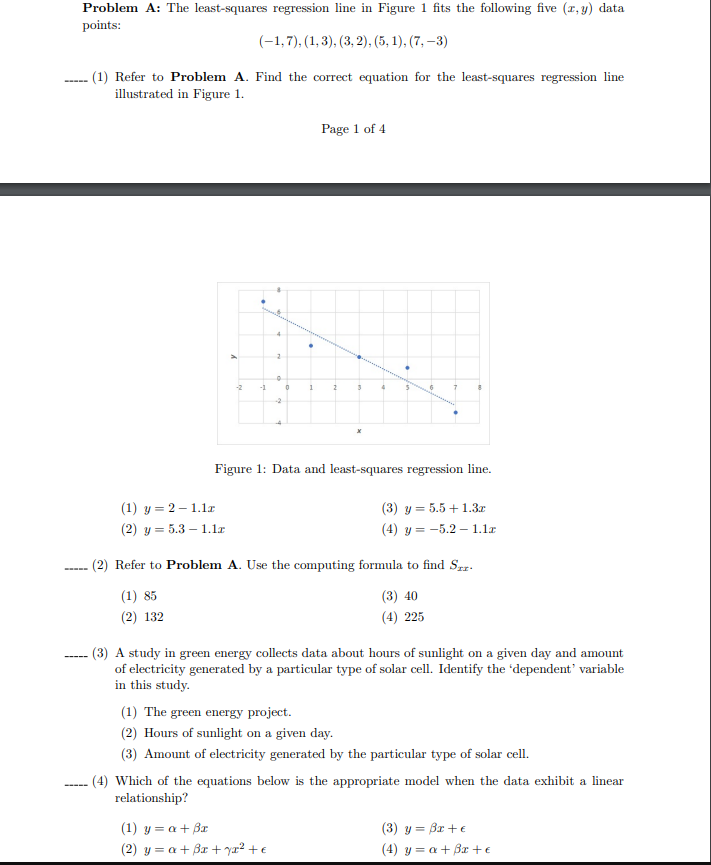Home / Expert Answers / Statistics and Probability / 1-7-1-3-3-2-5-1-7-3-1-refer-to-problem-a-find-the-correc-pa774

# (Solved): $(-1,7),(1,3),(3,2),(5,1),(7,-3)$ (1) Refer to Problem A. Find the correc ...???????

$(-1,7),(1,3),(3,2),(5,1),(7,-3)$ (1) Refer to Problem A. Find the correct equation for the least-squares regression line illustrated in Figure 1. Page 1 of 4 Figure 1: Data and least-squares regression line. (1) $$y=2-1.1 x$$ (3) $$y=5.5+1.3 x$$ (2) $$y=5.3-1.1 x$$ (4) $$y=-5.2-1.1 x$$ (2) Refer to Problem A. Use the computing formula to find $$S_{x x}$$. (1) 85 (3) 40 (2) 132 (4) 225 (3) A study in green energy collects data about hours of sunlight on a given day and amount of electricity generated by a particular type of solar cell. Identify the 'dependent' variable in this study. (1) The green energy project. (2) Hours of sunlight on a given day. (3) Amount of electricity generated by the particular type of solar cell. (4) Which of the equations below is the appropriate model when the data exhibit a linear relationship? (1) $$y=\alpha+\beta x$$ (3) $$y=\beta x+\epsilon$$ (2) $$y=\alpha+\beta x+\gamma x^{2}+\epsilon$$ (4) $$y=\alpha+\beta x+\epsilon$$

We have an Answer from Expert